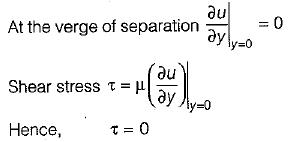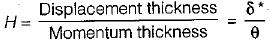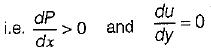Courses

# Test: Boundary Layer Theory - 2

## 10 Questions MCQ Test Topicwise Question Bank for Mechanical Engineering | Test: Boundary Layer Theory - 2

Description
This mock test of Test: Boundary Layer Theory - 2 for GATE helps you for every GATE entrance exam. This contains 10 Multiple Choice Questions for GATE Test: Boundary Layer Theory - 2 (mcq) to study with solutions a complete question bank. The solved questions answers in this Test: Boundary Layer Theory - 2 quiz give you a good mix of easy questions and tough questions. GATE students definitely take this Test: Boundary Layer Theory - 2 exercise for a better result in the exam. You can find other Test: Boundary Layer Theory - 2 extra questions, long questions & short questions for GATE on EduRev as well by searching above.
QUESTION: 1

Solution:
QUESTION: 2

### It is recommended that the diffuser angle should be kept less than 18° because

Solution:

If diffuser angle is more, eddies formation may takes place and also flow separation may occurs.

QUESTION: 3

### Boundary layer separation is caused by

Solution:

QUESTION: 4

Laminar sub-layer acts as

Solution:

In turbulent flow analysis along the boundary layer, a thin layer of fluid in the immediate neighbourhood of the boundary where viscous shear stress is predominant while the shear stress due to turbulence is negligible. This portion is known as laminar sublayer.
In turbulent flow heat transfer is more as compared to laminar flow and also is this laminar sublayer heat transfer is very less while, it is present is turbulent flow hence it acts as insulating medium in turbulent flow.

QUESTION: 5

At the point of boundary layer separation

Solution:QUESTION: 6

If u = free stream velocity
u = local velocity.
δ = boundary layer thickness
Then the momentum thickness θ is given by

Solution:
QUESTION: 7

The energy thickness δe is given by

Solution:
QUESTION: 8

The displacement thickness δ* is

Solution:
QUESTION: 9

Given that
δ = boundary layer thickness
δ* = displacement thicknes
δe = energy thickness
θ = momentum thickness
The shape factor H is given by

Solution:

Shape factor is given byQUESTION: 10

Boundary layer flow separates from the surface if

Solution: